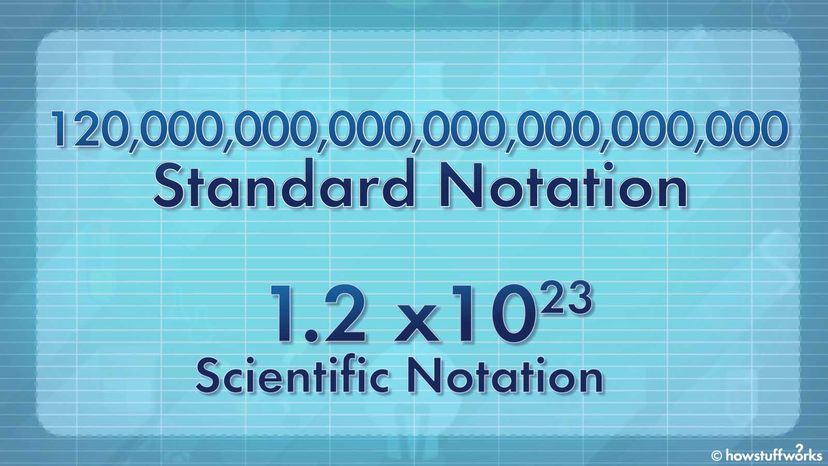# Scientific Notation Is Math's Version of ShorthandScientific notation helps you take absurdly large numbers, like 120 sextillion, and write them in what could be considered mathematical "shorthand." HowStuffWorks

Astronomers estimate there are at least 120 sextillion stars in the observable universe. By most accounts, that's a seriously impressive number. One sextillion is written out as a "1" followed by 21 zeros. And when we commit 120 sextillion to paper numerically, it looks like this:

120,000,000,000,000,000,000,000

But Houston, we have a problem. Long strings of zeros and commas aren't exactly great reading material. Taken in context, this particular sum should make our jaws drop. Just think about its implications: There are more stars in the universe than there are grains of sand in all of Earth's beaches and deserts — or cells in the human body. Truly, 120 sextillion is a mind-blowing number.

Yet comprehension is the key to communication. The plain fact is, one sextillion — or 1,000,000,000,000,000,000,000 — isn't a sum most of us think about or interact with every day. So its significance is hard to grasp. Besides, all those lined-up zeros look rather dull, and writing them out by hand or keyboard is a tedious, error-prone chore.

Now wouldn't it be great if there was some kind of useful shorthand? Well fortunately, there is. Ladies and gents, let's talk about scientific notation.

Contents

## The Basics of Scientific Notation

As any bank teller should know, 100 is equal to 10 x 10. But instead of writing "10 x 10" out, we could save ourselves some ink and write 102 instead.

What's that itty-bitty "2" next to the number 10? We're glad you asked. That's what's called an exponent. And the full-sized number (i.e., 10) to its immediate left is known as the base. The exponent tells you how many times you need to multiply the base by itself.

So 102 is just another way of writing 10 x 10. Similarly, 103 means 10 x 10 x 10, which equals 1,000.

(By the way, when solving math problems on a computer or calculator, the caret symbol — or ^ — is sometimes used to denote exponents. Hence, 102 can also be written as 10^2, but we'll save that conversation for another day.)

Scientific notation relies on exponents. Consider the number 2,000. If you wanted to express this sum in scientific notation, you'd write 2.0 x 103.

Here's how we made that conversion. When you use scientific notation, what you're really doing is taking a small number (i.e., 2.0) and multiplying it by a specific exponent of 10 (i.e., 103).

To get the former, put a decimal point behind the first non-zero digit in the original number. Doing so in this example leaves us with "2.000." Mathematically that can also be written as just "2.0."

Obviously, 2.0 is much smaller than the 2,000 we started out with. But a careful count reveals there are three other digits (all zeros) behind the first digit in "2,000." That gives us our exponent value. So, what happens when we multiply 2.0 by 103 — or 10 x 10 x 10? Lo and behold, we end up with the same sum we started out with: 2,000. Hallelujah.

## A Sextillion by Another Name

All right, time to have some fun. Through the steps we outlined above, we can use scientific notation to express 4,000 as 4.0 x 103. Likewise, 27,000 becomes 2.7 x 104 and 525,000,000 turns into 5.25 x 108.

Ah but dare we convert 120 sextillion, that giant, unwieldy number from our opening sentence? Indeed, we do. Take a good, hard look at 120,000,000,000,000,000,000,000.

Altogether, there are 23 digits behind the "1." (Go ahead and count 'em up. We'll wait.) Ergo, in scientific notation, 120,000,000,000,000,000,000,000 is expressed as 1.2 x 1023.

But admit it, the latter is way easier on the eyes. Besides, the exponent gives you an immediate sense of how ginormous the total number really is. And it does so in a way that tallying up the zeros never could. Such is the simplifying beauty of scientific notation.

## Going Negative

You'll be happy to know this process can be applied to numbers that are smaller than one.

Suppose you've only got one-tenth of an apple. Mathematically that means you have 0.10 apples at your disposal. Likewise, if there's only one-millionth of an apple on your lunch tray, you're dealing with a paltry 0.000001 apples. Tough break.

There's a way to write this sum down using scientific notation — and it's not all that different from the technique we've been practicing.

Here (again) we'll need to take the existing decimal point and put it to the right of the number's first non-zero digit. Do that and you'll wind up with a plain old "1." In the name of mathematical clarity, we'll write this as "1.0."

OK, so in order to get 0.000001, we'll need to multiply our 1.0 by another exponent of 10. But here's the twist: The exponent will be a negative number.

Take another gander at 0.000001. See how there are six digits behind the decimal point? That forces us to multiply our 1.0 by 10-6. So in summary, 1.0 x 10-6 is how we express one-millionth, or 0.000001, in scientific notation.

By the same token, 6.0 x 10-3 means 0.006. Accordingly, 0.00086 would be written as 8.6 x 10-4. And so on. Happy calculating.